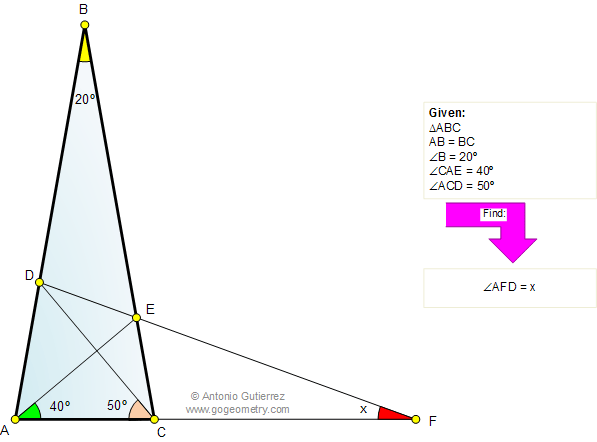Problem 691: Isosceles Triangle, 80-80-20 Degrees, Angles, Congruence The figure shows an isosceles triangle ABC with AB = BC and angle B = 20°. Points D and E are on AB and BC, respectively so that the measure of angle CAE is 40 degrees and the measure of angle ACD is 50 degrees. DE and AC meet at F. Find the measure of angle AFD.How to Solve It, Interactive Mind Map George Pólya's 1945 book "How to Solve It, A new aspect of Mathematical Method", is a book describing methods of problem solving. It suggests the following steps when solving a mathematical problem: (1) First, you have to understand the problem. (2) After understanding, then make a plan. (3) Carry out the plan. (4) Look back on your work. How could it be better?    Mind Map Help. To see a note: Hover over a yellow note button. To Fold/Unfold: click a branch. To Pan: click and drag the map canvas.Recent Additions